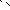# MINLPLib

### A Library of Mixed-Integer and Continuous Nonlinear Programming Instances

#### Instance ex9_2_2

 Formatsⓘ ams gms lp mod nl osil pip py Primal Bounds (infeas ≤ 1e-08)ⓘ 99.99996939 p1 ( gdx sol ) (infeas: 3e-11) Other points (infeas > 1e-08)ⓘ Dual Boundsⓘ 99.99992444 (ANTIGONE)99.99996919 (BARON)99.99996939 (COUENNE)99.99996939 (GUROBI)99.99996939 (LINDO)99.99996939 (SCIP) Referencesⓘ Floudas, C A, Pardalos, Panos M, Adjiman, C S, Esposito, W R, Gumus, Zeynep H, Harding, S T, Klepeis, John L, Meyer, Clifford A, and Schweiger, C A, Handbook of Test Problems in Local and Global Optimization, Kluwer Academic Publishers, 1999.Shimizu, K and Aiyoshi, E, A New Computational Method for Stackelberg and Min-Max Problems by Use of a Penalty Method, IEEE Transactions on Automatic Control, 26:2, 1981, 460-466. Sourceⓘ Test Problem ex9.2.2 of Chapter 9 of Floudas e.a. handbook Added to libraryⓘ 31 Jul 2001 Problem typeⓘ QCQP #Variablesⓘ 10 #Binary Variablesⓘ 0 #Integer Variablesⓘ 0 #Nonlinear Variablesⓘ 10 #Nonlinear Binary Variablesⓘ 0 #Nonlinear Integer Variablesⓘ 0 Objective Senseⓘ min Objective typeⓘ quadratic Objective curvatureⓘ convex #Nonzeros in Objectiveⓘ 2 #Nonlinear Nonzeros in Objectiveⓘ 2 #Constraintsⓘ 11 #Linear Constraintsⓘ 7 #Quadratic Constraintsⓘ 4 #Polynomial Constraintsⓘ 0 #Signomial Constraintsⓘ 0 #General Nonlinear Constraintsⓘ 0 Operands in Gen. Nonlin. Functionsⓘ Constraints curvatureⓘ indefinite #Nonzeros in Jacobianⓘ 24 #Nonlinear Nonzeros in Jacobianⓘ 8 #Nonzeros in (Upper-Left) Hessian of Lagrangianⓘ 10 #Nonzeros in Diagonal of Hessian of Lagrangianⓘ 2 #Blocks in Hessian of Lagrangianⓘ 6 Minimal blocksize in Hessian of Lagrangianⓘ 1 Maximal blocksize in Hessian of Lagrangianⓘ 2 Average blocksize in Hessian of Lagrangianⓘ 1.666667 #Semicontinuitiesⓘ 0 #Nonlinear Semicontinuitiesⓘ 0 #SOS type 1ⓘ 0 #SOS type 2ⓘ 0 Minimal coefficientⓘ 1.0000e+00 Maximal coefficientⓘ 1.0000e+01 Infeasibility of initial pointⓘ 60 Sparsity JacobianⓘSparsity Hessian of Lagrangianⓘ```\$offlisting
*
*  Equation counts
*      Total        E        G        L        N        X        C        B
*         12        9        0        3        0        0        0        0
*
*  Variable counts
*                   x        b        i      s1s      s2s       sc       si
*      Total     cont   binary  integer     sos1     sos2    scont     sint
*         11       11        0        0        0        0        0        0
*  FX      0
*
*  Nonzero counts
*      Total    const       NL      DLL
*         27       17       10        0
*
*  Solve m using NLP minimizing objvar;

Variables  objvar,x2,x3,x4,x5,x6,x7,x8,x9,x10,x11;

Positive Variables  x2,x3,x4,x5,x6,x7,x8,x9,x10,x11;

Equations  e1,e2,e3,e4,e5,e6,e7,e8,e9,e10,e11,e12;

e1.. x2*x2 + (-10 + x3)*(-10 + x3) - objvar =E= 0;

e2..    x2 =L= 15;

e3..  - x2 + x3 =L= 0;

e4..  - x2 =L= 0;

e5..    x2 + x3 + x4 =E= 20;

e6..  - x3 + x5 =E= 0;

e7..    x3 + x6 =E= 20;

e8.. x4*x8 =E= 0;

e9.. x5*x9 =E= 0;

e10.. x6*x10 =E= 0;

e11.. x7*x11 =E= 0;

e12..    2*x2 + 4*x3 + x8 - x9 + x10 =E= 60;

* set non-default bounds
x4.up = 20;
x5.up = 20;
x6.up = 20;
x7.up = 20;
x8.up = 20;
x9.up = 20;
x10.up = 20;
x11.up = 20;

Model m / all /;

m.limrow=0; m.limcol=0;
m.tolproj=0.0;

\$if NOT '%gams.u1%' == '' \$include '%gams.u1%'

\$if not set NLP \$set NLP NLP
Solve m using %NLP% minimizing objvar;

```

Last updated: 2023-08-16 Git hash: 2519540e